# All Categories Meeting a Threshold

This module will walk you through writing a text insight listing out all the categories or values in your dashboard that meet some sort of threshold you set. In this example, we'll be writing this sentence:

`A states have more than \$B in loans that are more than 90 days overdue, most notably C, D, and E.`

🚧

Thinks to keep in mind while working through this module

• If there are a lot of categories that meet the threshold, how many do you want to list in your narrative?
• What if there are no categories that meet the threshold?

## Calculations

For this insight, we need to know the threshold that determines which categories to list in our narrative. That threshold can be a fixed value, like if something is a positive number. Or it can by a dynamic value based on the data in the dashboard, like the average. We also need to know the number of categories that meet that threshold and what the actual categories are. Let's start by pulling the threshold out of the data, which I want to be the average 90-day loan value across all states. That way, I can list out how many states have above-average risky loans.

Use the Hidden Sheet Full worksheet for these calculations. Using this worksheet means the data won't change as the audience interacts with the dashboard.

## Calc: 90-day loan average amount##

Step 1: Filter
We just want 90-day loans, so the first step is to filter the table to only include data when the "days overdue bin" column is equal to a value of 3. This will give us a data table with just 90-day loans.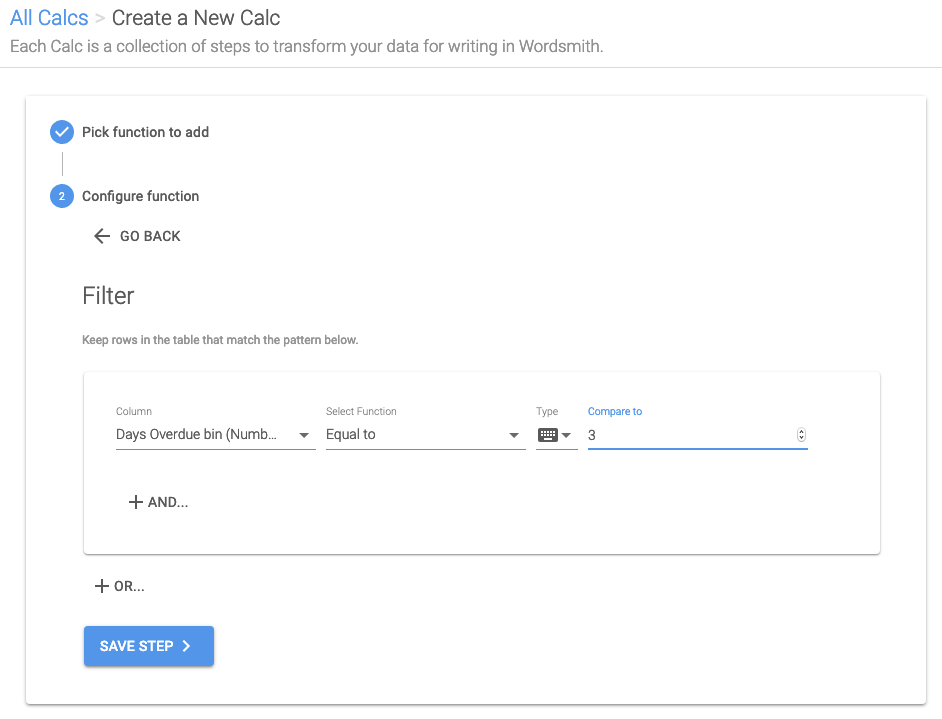Step 2: Group
We're comparing these loans on the state level, so the next step is to group up the 90-day loan amounts for each state.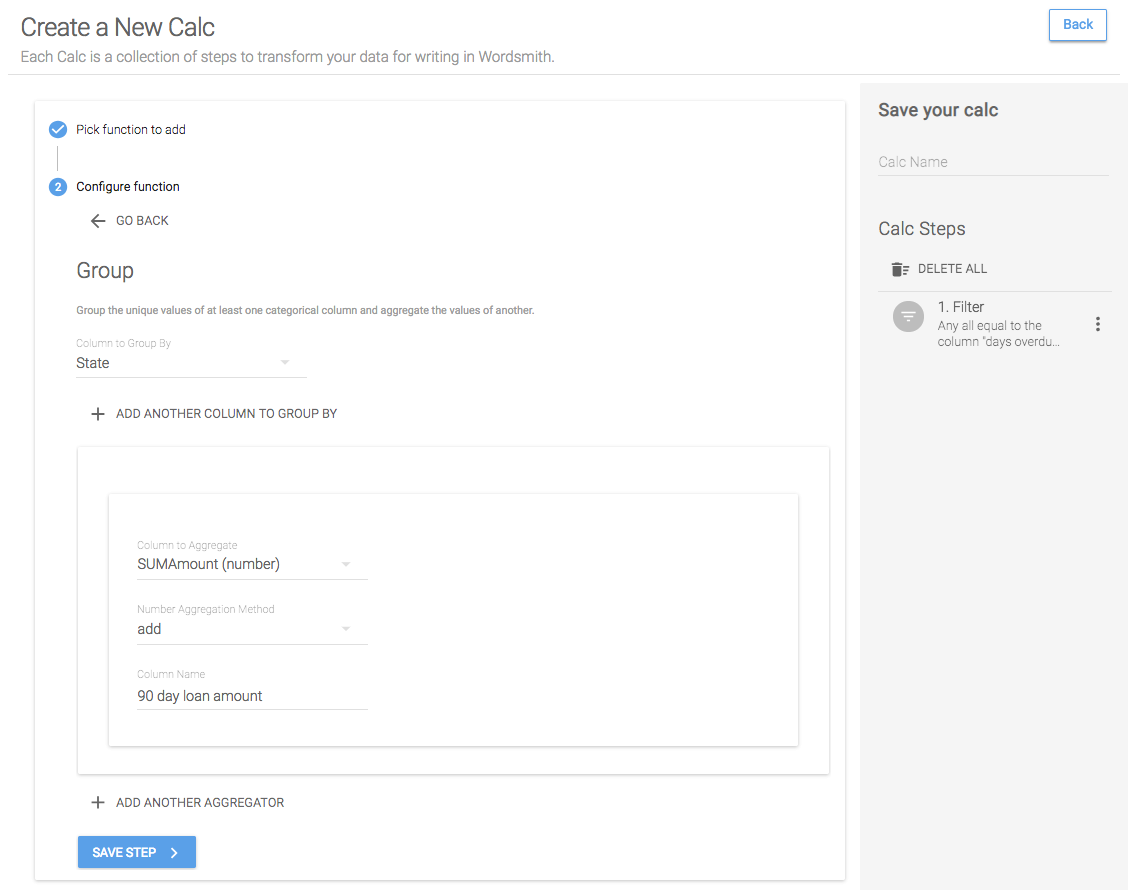Step 3: Aggregate
Now that we have just the 90-day loans for each state, we need the average of all of them. So we'll aggregate the SUMAmount column in the table by taking the average of all the values. Because we're creating a new column in the table, we're prompted to give this column a name.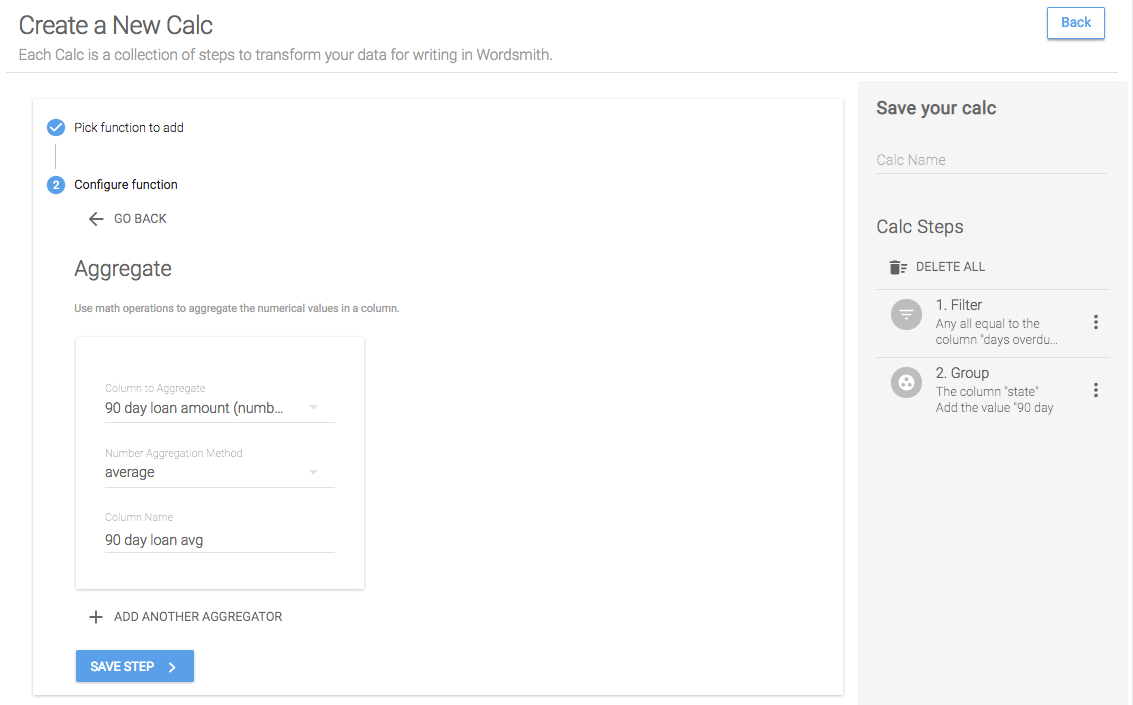Save Calc
Now we have our table transformed to just the data we care about, in this case just the average of the 90-day loans. So we'll name this calc and save it.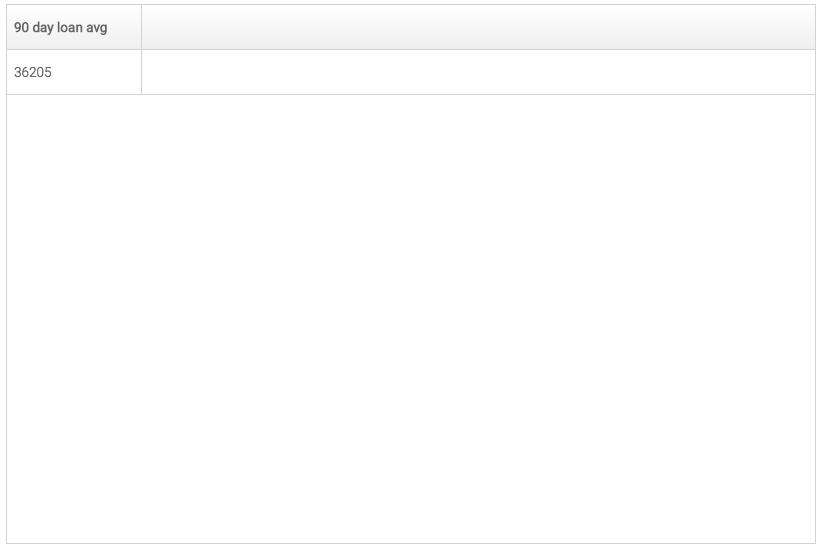## Calc: States with 90-day loan amounts higher than the average##

Step 1: Filter
Again, since we only care about the 90-day loans, we'll start by filtering this table down to just the 90-day loans.Step 2: Group
We're comparing these loans on the state level, so the next step is to group up the 90-day loan amounts for each state.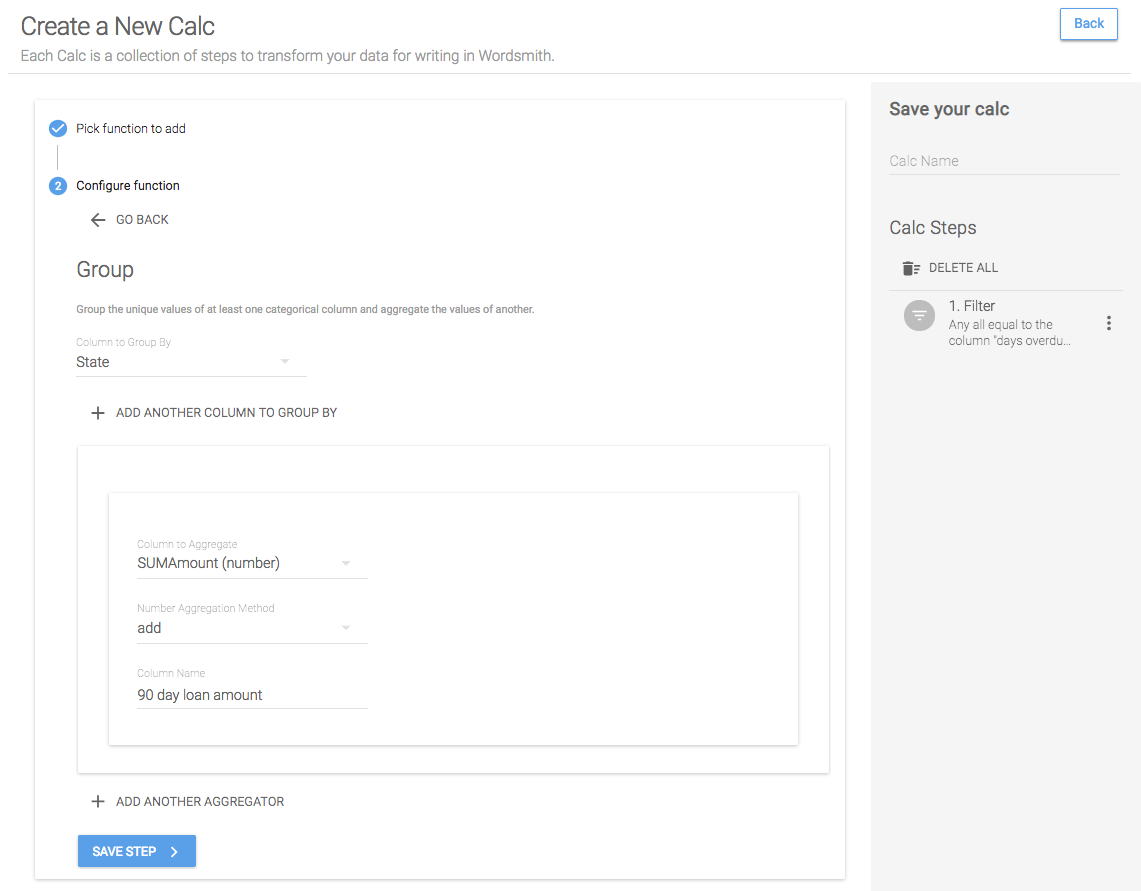Step 3: Filter
Now that we have the 90-day loan amount for each state, let's filter this table down to just the states that are higher than the average we pulled in the first calc. To do that, we'll select the column we want to compare, which is the "90 day loan amount" column we just created by aggregating. Then we'll select the function we want to use, which in this case will be "greater than". And we can change the type of data we're comparing from "column" to "calc". When we do this, we'll see all the calcs we've created of the same type as the column we've selected to compare them to (i.e. if the column we selected was text, we would just see the text calcs). We'll select the calc we created for the 90-day loan average and save this step.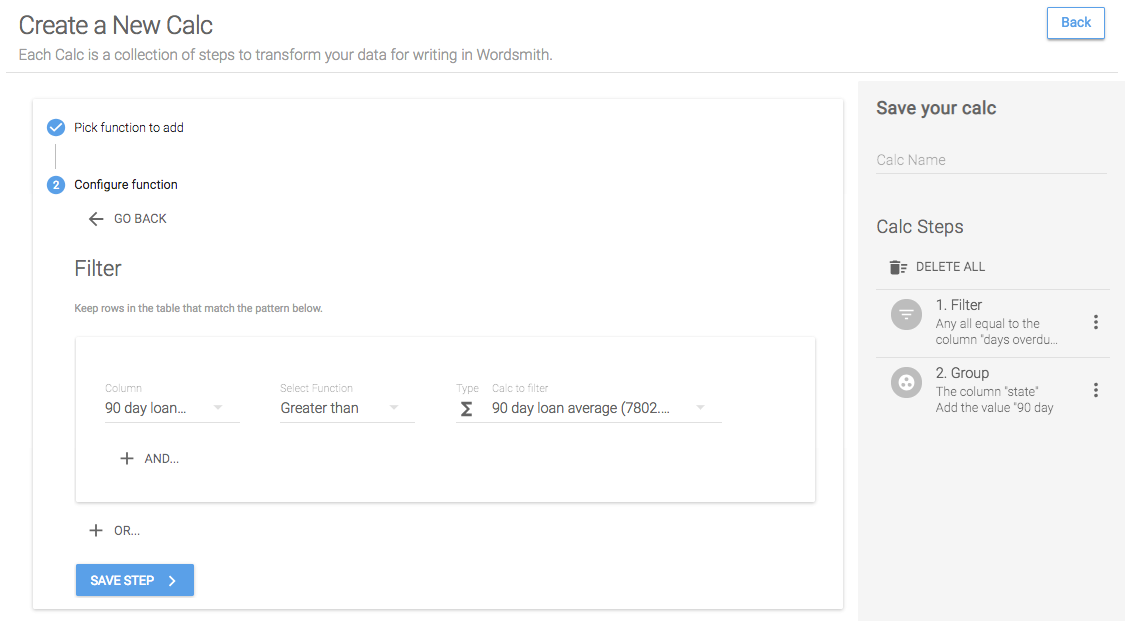Step 4: Sort
When we write about these states in our narrative, we'll want to have the highest states first, since those are the riskiest. So we'll sort this table in descending order to get the states with the most 90-day loans at the top.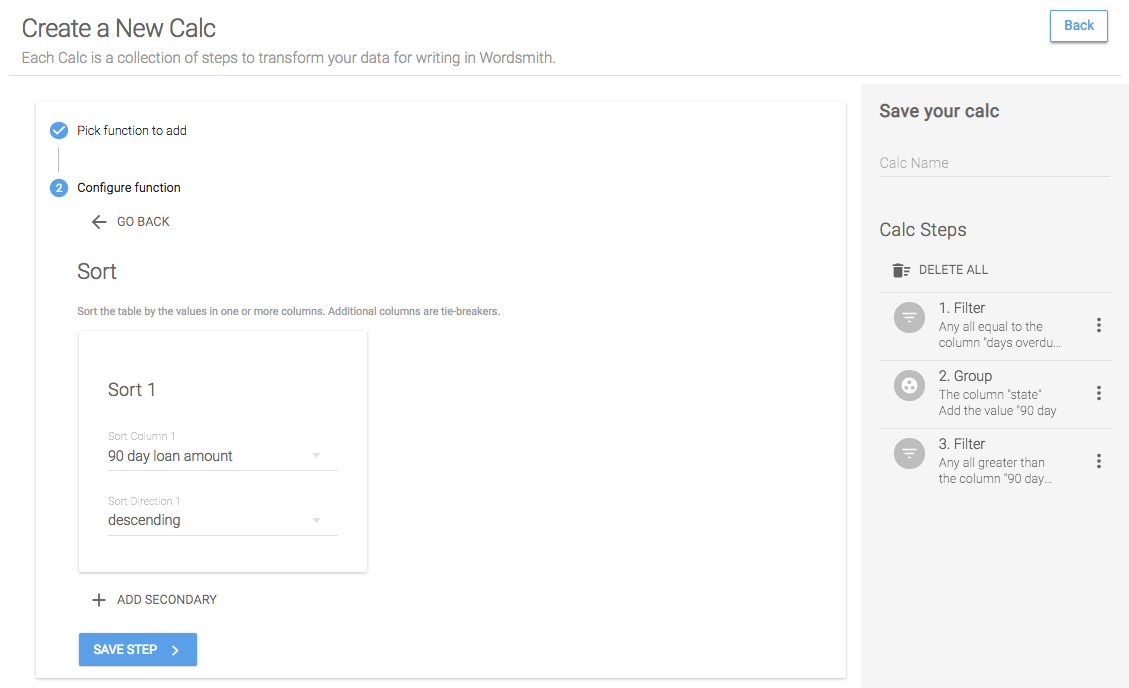Save Calc
Now when we save this calc, we'll have two Data Variables for the columns included in the table: state and 90 day loan amount. If there are multiple rows in the table, the values in each row are separated by a comma and the data type is a List.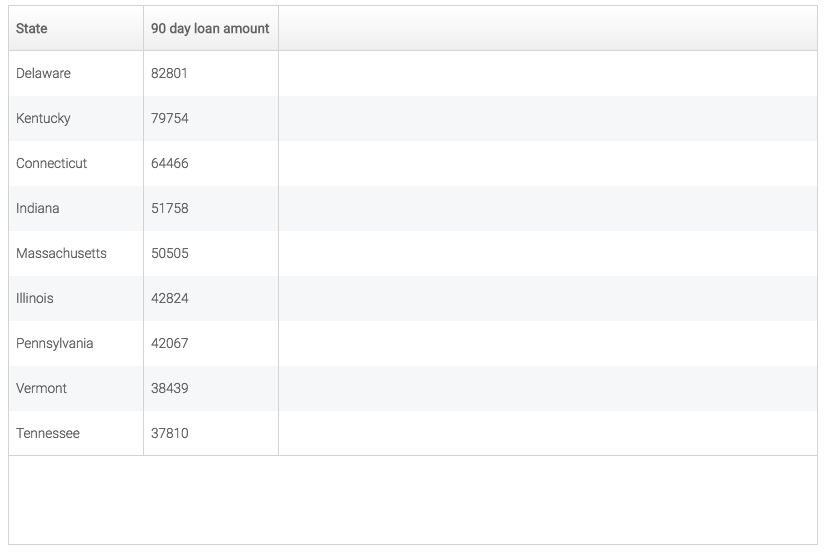## Template

Now that we have transformed the data by writing calculations, we have most of the Data Variables we need to write our sentence. But we don't yet have the number of states that have met our threshold. We could write another calc to aggregate the states by counting them instead of adding them, or we could write a Formula in our Template.

## Creating a Formula##

📘

A Formula is a new Data Variable created in the Template using other Data Variables. Use the drop-down in the Formula window to select Data Variables and see the functions available for them.

When you click on the 'Insert Data' button in the Template, you'll see all of the Data Variables you've created by writing calcs. In this example, I want to know the number of states that are included in the states above average Data Variable and I already have a Data Variable that's a list of all the states that are above average. So we'll create a Formula that returns the number of states in the list. When you click 'Create Formula', select the Data Variable "states above avg in 90 day loans 90 day loan amount" and choose the count function. Give this Formula a name and save it. Now we can use it just like any other Data Variable in the Template.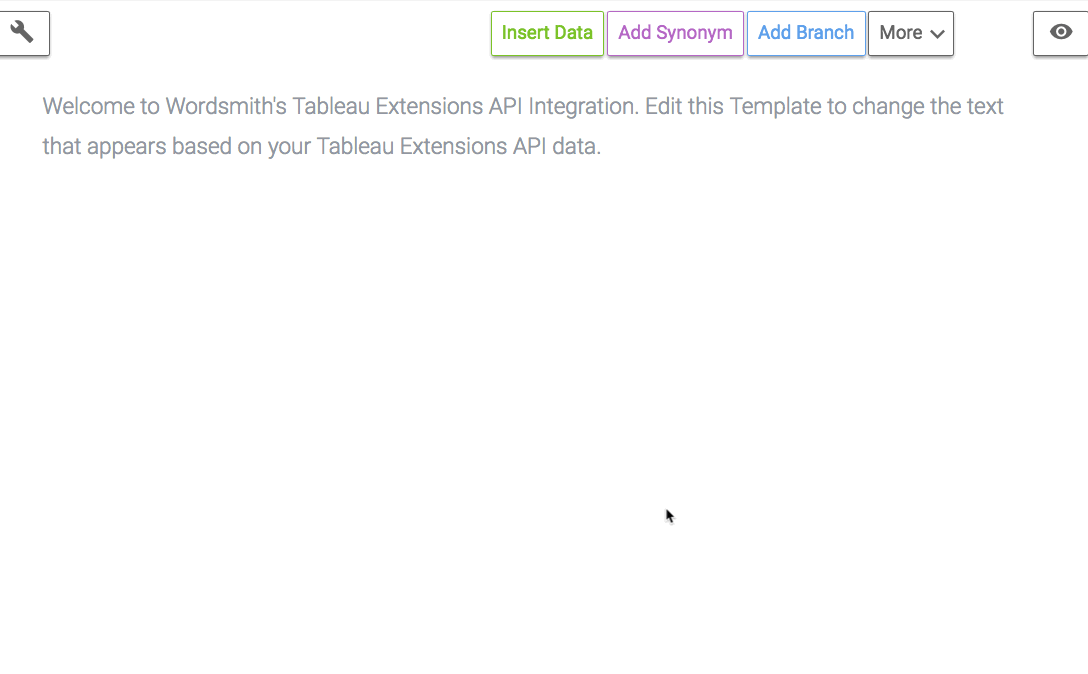## Inserting Data##

Now that we have all the Data Variables we need, the average 90-day loan amount, the states with loan amounts greater than that average, the 90-day loan amounts for those states, and the number of states, we can write our sentence. As we write, we'll insert the Data Variables we've created so that the data in our narrative reflects the current data in the dashboard.

As you insert the Data Variables, you'll see different formatting options for each based on the data type. For Lists, we can choose how many items to include in our sentence by changing the 'List Settings'.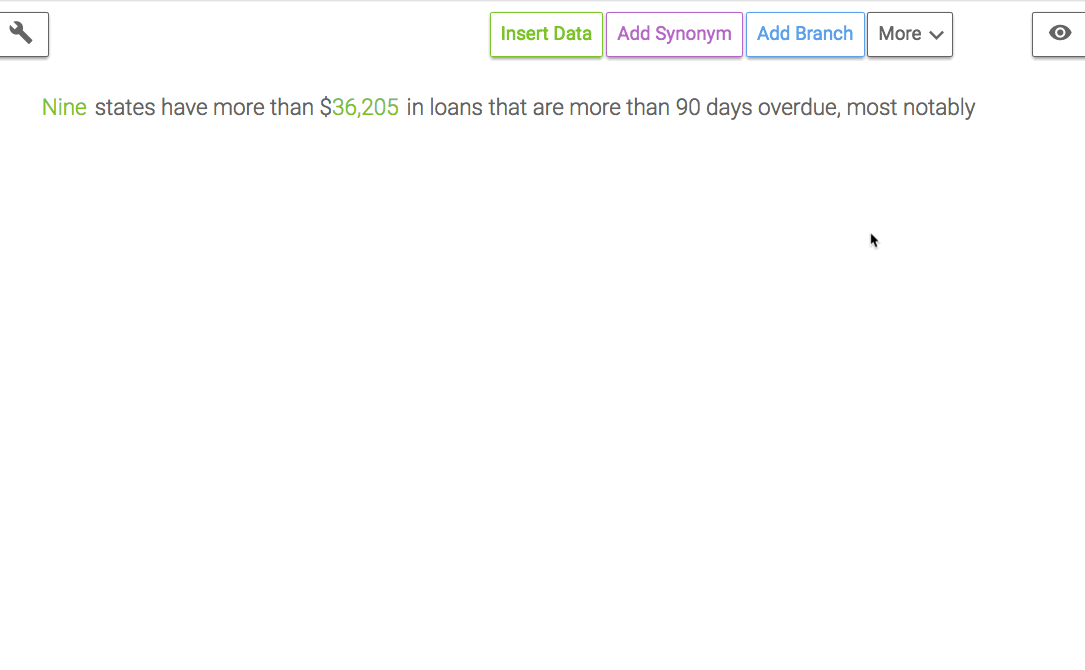🚧

By changing the 'List Settings' for the states Data Variable, we're accounting for the scenario where there are a lot of categories that meet the threshold. In this example, we'll only list the top 3.

We'll add a Branch to the Template to change the text based on that data so that if there are no categories that meet the threshold or a lot of categories, we'll acknowledge it. In this example, I want to change the entire sentence. But there are many other times when you'll just need to change one or two words, so you'll only need to put a Branch around those words.

Rule 1: If there are states that meet the threshold
When writing Branches, you need to account for every scenario in the data. And in this example, we want to only write the sentence if there are categories that meet the threshold. So the logic we write will be checking that the count of states formula is greater than 0. If it is, we'll write our sentence. And if it's not, we won't write anything because we only have one rule in the Branch.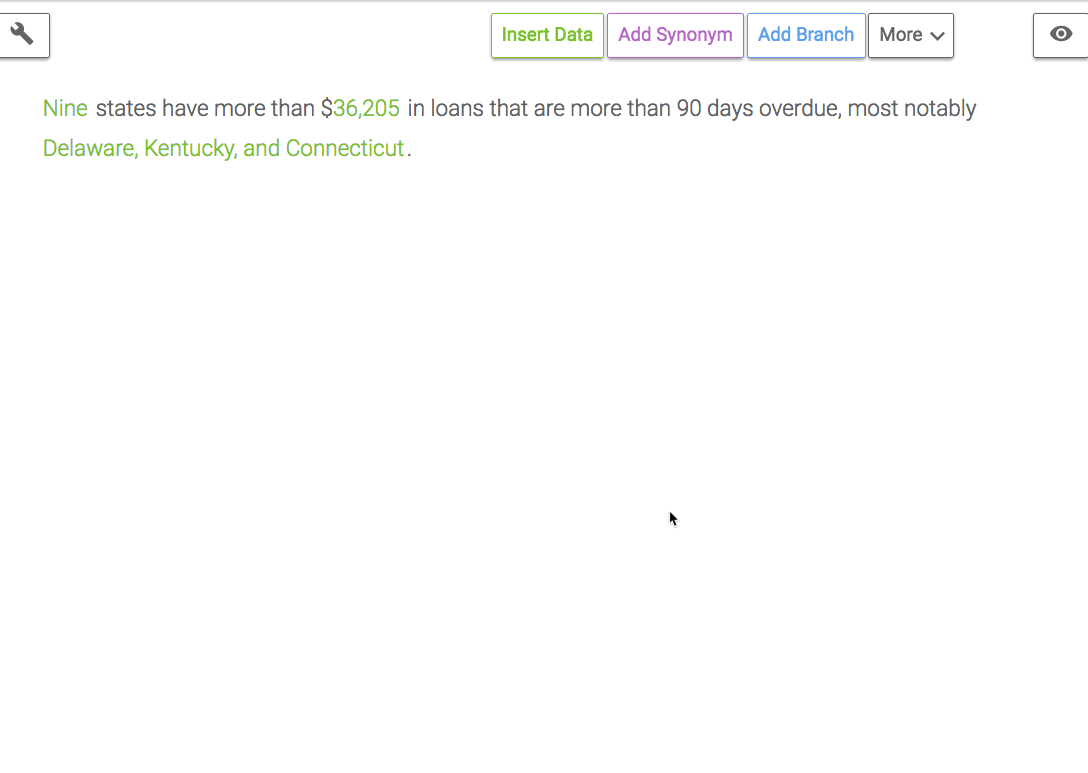🚧

By writing this Branch, the sentence will only be written if there are states that meet the threshold. And in case no states meet the threshold, nothing will be written.

## Nested Branches##

To account for a scenario where there are only threes states that meet the threshold, we'll add another Branch around the phrase " , most notably". If there are only three states and we list all of them, then it doesn't make sense to include that phrase.

Rule 1: There are more than three states meeting the threshold
We're writing just the first three states in the sentence, so we should only write ", most notably" if we didn't include all of the states, meaning there are more than three.

Default Rule
By selecting "Add text if no rules are true", you can create a default rule. This text is written if none of the rules in the Branch are true. In this example, if none of the rules in our Branch are true, then we know there are no more than three states meeting the threshold, so we can use a colon and list them all.## Dashboard

Refresh the narrative in Tableau to pull this insight into your dashboard.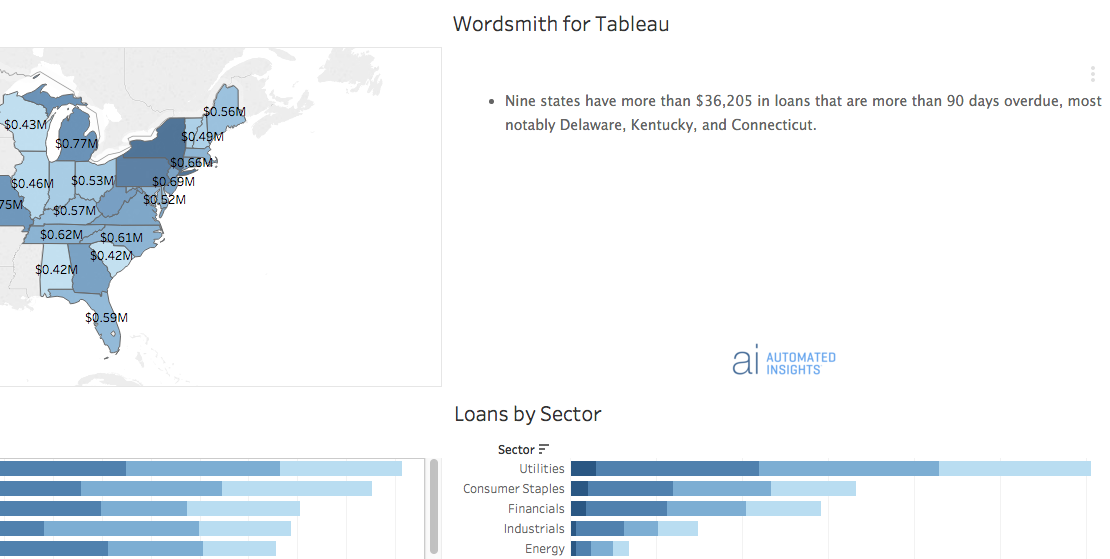👍

Check out the next module to write a context-dependent drill down insight.